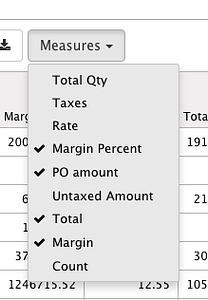# Additional measure in Odoo graph viewThis article will help you to add measures in graph view, my exemple is based on sale order model, for all additional computed fields, attribute store must be set to True, otherwise you won’t be able to choose it from the dropdown list measure in graph view. When grouping, you might want to calculate on some fields the average instead of the sum, if so, you need to use `group_operator = 'avg'` directly in your model file, see `margin_percent` field bellow.

```import logging
import openerp.addons.decimal_precision as dp
from datetime import datetime, date, timedelta
from openerp.exceptions import except_orm, Warning, RedirectWarning
from openerp import models, fields, api, _
from openerp.osv import osv

_logger = logging.getLogger(__name__)

class SaleOrder(models.Model):
_inherit = "sale.order"

@api.depends('name','purchase_order_ids.amount_untaxed')
def _compute_purchase_order_ids(self):
for rec in self:
ids = []
total = 0.0
purchase_order_ids = self.env['purchase.order'].search([('origin','=', rec.name)])
for po in purchase_order_ids:
ids.append(po.id)
total += po.amount_untaxed
if len(ids) > 0:
rec.update({
'purchase_order_ids':[[6,0,ids]],
'total_purchase_order_amount':total,
})

@api.depends('amount_untaxed','total_purchase_order_amount')
def _compute_margin(self):
for rec in self:
rec.update({
'margin': rec.amount_untaxed - rec.total_purchase_order_amount
})

@api.depends('amount_untaxed','margin')
def _compute_margin_percent(self):
for rec in self:
margin_percent = 0.0
_logger.critical(rec.amount_untaxed)
if rec.amount_untaxed > 0.0:
margin_percent = rec.margin * 100 / rec.amount_untaxed
rec.update({
'margin_percent': margin_percent
})

purchase_order_ids = fields.Many2many(comodel_name='purchase.order', string='Purchase Orders', compute=_compute_purchase_order_ids)
total_purchase_order_amount = fields.Float(string='PO amount',store=True, compute=_compute_purchase_order_ids)
margin = fields.Float(string='Margin',store=True, compute=_compute_margin)
margin_percent = fields.Float(string='Margin Percent',group_operator = 'avg', store=True, compute=_compute_margin_percent)
```

Find the view_id of your graph view and inherit from it. then place your new field as measure using as usual xpath.

```<record model="ir.ui.view" id="sale_order_graph_view">
<field name="name">Sale Order Graph</field>
<field name="model">sale.order</field>
<field name="inherit_id" ref="sale.view_sale_order_graph" />
<field name="arch" type="xml">
<data>
<xpath expr="//field[@name='amount_total']" position="after">
<field name="total_purchase_order_amount" type="measure" />
<field name="margin" type="measure" />
<field name="margin_percent" type="measure" />
</xpath>
</data>
</field>
</record>
```### 2 thoughts on “Additional measure in Odoo graph view”

1.•